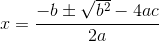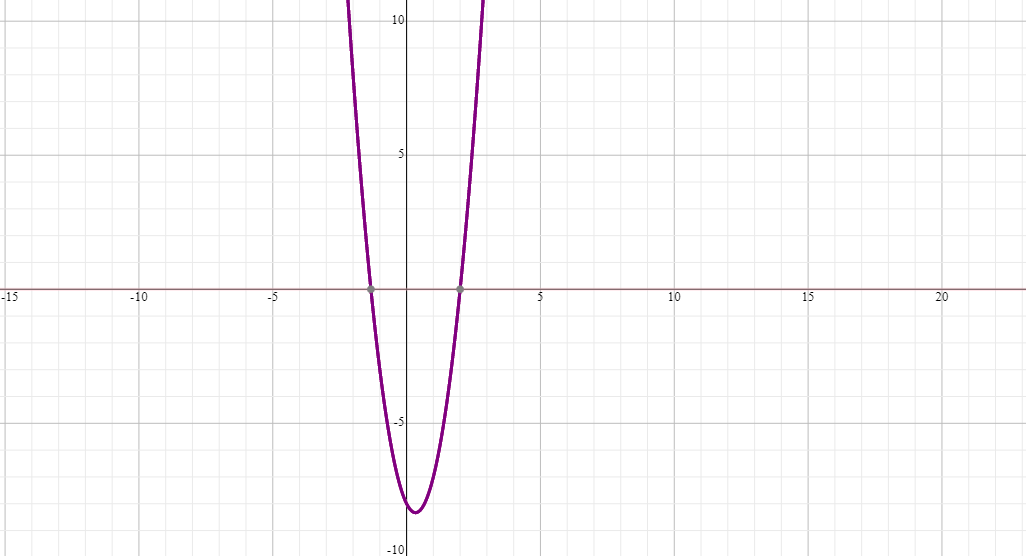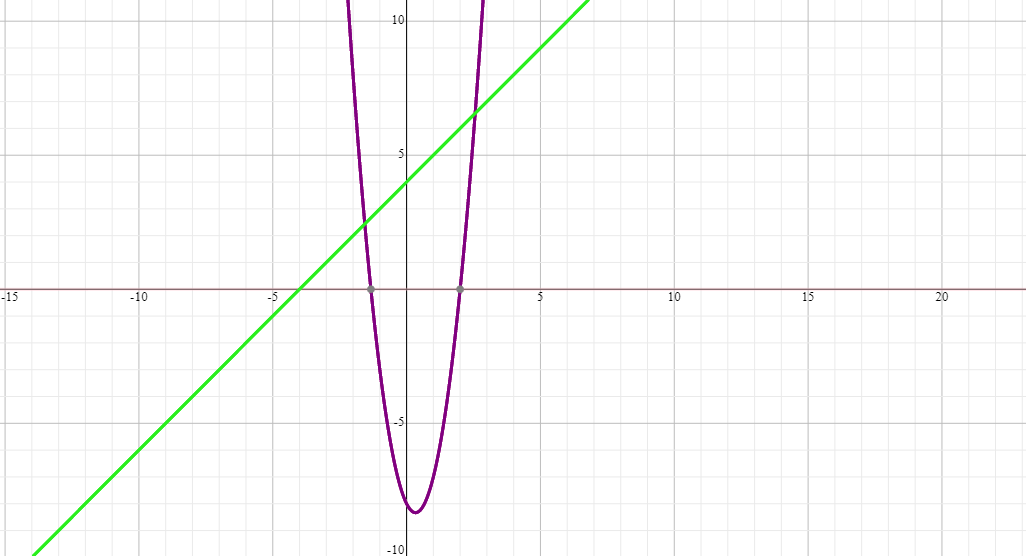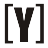# Algebra

## 1. Mathematical Sentence

Mathematical sentences include:

 Type of Sentences Example Correct sentence 2 + 3 = 5 3 x 0² – 2 x – 8 = 0 x = 2 or x = -1⅓ Wrong sentence 5 – 3 = 3 12 + 4x – x² = (2 + x) (x – 5) Open Sentence x + 2 = 6 y = x² – 4 similarities are open sentences that contain signs of similarity 6 + 2 = 8 12 – 6 = 6 Inequality is a mathematical sentence that uses the sign of inequality 4 < 6 24 + 4 > 20 + 2 6 ≠ 4 Equation open sentence which states the relationship “equal to” x + 3 = 5 2 x² – 7x = 15 Inequality open sentences that use a sign of inequality and contain variables x + 3 < 6 (x – 5) (x – 8) ≤ 0 3 + 5x – 2x² > 0

## 2. Factorial

A. by finding equation factor

Example:
6a³b – 2a² b + 8 ab = 2 ab (3a² – a + 4)
2 ab is equation factor

B. Collecting and Grouping

Example:
2px – 3qy – qx + 6py =
(2px – qx) + (6py – 3qy) =
x (2 p – q) + 3 y (2p – q) =
(2 p – q) (x + 3y)

C. Identity elements
a² – b² = (a + b)(a – b)

Example:
16 c² – 9 d² = 4²c² – 3²d²
= (4c + 3d)(4c – 3d)

Example: x² + 5x + 6

Result of times 6x²
Total 5x
Obtained: 2x + 3x

x² + 5x + 6 can be converted into x² + 2x + 3x + 6
= (x² + 2x) + (3x + 6)
= x (x + 2) + 3 (x + 2)
= (x + 3)(x + 2)

ax² + bx + c = 0 with a ≠ 0
a, b, c ϵ R

The solution can be done with:
a. Factorial

Example:
2a² – 5a + 3 = 0
2a² – 2a – 3a + 3 = 0
(2a² – 2a) – (3a – 3) = 0
2a (a – 1) – 3(a – 1) = 0
(2a – 3)(a – 1) = 0
2a = 3 or a = 1
a = 1½ or a = 1

ax² + bx + c = 0
– move c to the right side
ax² + bx = -c
– add the two sections with (½ coefficient x)²
ax² + bx + (½b)² = -c + (½b)²
– then factorized

Example:
x² + 2x – 8 = 0
x² + 2x = 8
x² + 2x + (2/2)² = 8 + (2/2)²
x² + 2x + 1 = 8 + 1
(x + 1)² = 9
x + 1 = √9
x + 1 = ± 3
x = 2 or x = -4

c. Using Formulad. Using Graph
for Example:
3x² – 2x – 8 = 0

this problem can be solved in 2 method:

First method
y = 3x² – 2x – 8

 x -1 0 1 2 y -3 -8 -7 0from graph x = -1⅓ or 2

Second Method
3x² – 2x – 8 = 0
3x² = 2x + 8
y1 = 3x²

 x -2 -1 0 1 2 y 12 3 0 3 12

y2 = 2x + 8

 x -1 0 1 y 6 8 10from graph x = -1⅓ or 2YoosFuhl.com Would you like to receive notifications on latest updates? No Yes